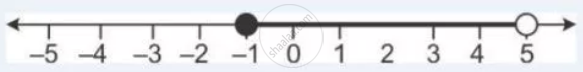Share

# Given X ∈ {Real Numbers}, Find the Range of Values of X for Which -5 ≤ 2x – 3 < X + 2 and Represent It on a Number Line. - Mathematics

Course

#### Question

Given x ∈ {real numbers}, find the range of values of x for which -5 ≤ 2x – 3 < x + 2 and represent it on a number line.

#### Solution

-5 ≤ 2x – 3 < x + 2

-5 ≤ 2x – 3 and 2x – 3 < x + 2

-2 ≤ 2x and x < 5

-1 ≤ x and x < 5

Required range is -1 ≤ x < 5.

The required graph is:Is there an error in this question or solution?